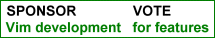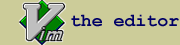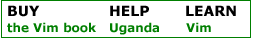Efficient python folding : Fold python code nicely and toggle with one keystroke

 created by Daniel Nogradi script type ftplugin description Folding goes like this: 1. Only top level class or function definitions are folded (no nesting) 2. Folding is done one line after the class or function definition, so     for example the line 'class foo( bar )' is right above the fold 3. Fold text is the first line of the corresponding docstring (if any)     together with the number of folded lines 4. Toggle all folds on/off with the key F 5. Toggle the fold under the cursor on/off with the key f 6. In some rare cases folding can break down which can be fixed by :call ReFold()     The reason for this break down is not known sometimes it happens when jumping     between different files using tags. In addition the script binds the key (hint: _e_xecute) to saving the file and executing it in the interpreter assuming that /usr/bin/env exists otherwise you need to change this key mapping slightly. The keys 'gd' (hint: _g_o _d_efinition) are also bound to look for the definition of a function under the cursor similarly to the same key binding for C. Inspired by vimscript #515, actually the way the number of lines are displayed is stolen from there :). A related script is vimscript #781 and a tip on toggling a fold is vimtip #108. The content of the script is this, in case you find it more convenient to copy/paste it than downloading: " Only do this when not done yet for this buffer if exists("b:did_ftplugin") finish endif let b:did_ftplugin = 1 map :w:!/usr/bin/env python % map gd /def set foldmethod=expr set foldexpr=PythonFoldExpr(v:lnum) set foldtext=PythonFoldText() map f za map F :call ToggleFold() let b:folded = 1 function! ToggleFold()     if( b:folded == 0 )         exec "normal! zM"         let b:folded = 1     else         exec "normal! zR"         let b:folded = 0     endif endfunction function! PythonFoldText()     let size = 1 + v:foldend - v:foldstart     if size < 10         let size = " " . size     endif     if size < 100         let size = " " . size     endif     if size < 1000         let size = " " . size     endif          if match(getline(v:foldstart), '"""') >= 0         let text = substitute(getline(v:foldstart), '"""', '', 'g' ) . ' '     elseif match(getline(v:foldstart), "'''") >= 0         let text = substitute(getline(v:foldstart), "'''", '', 'g' ) . ' '     else         let text = getline(v:foldstart)     endif          return size . ' lines:'. text . ' ' endfunction function! PythonFoldExpr(lnum)     if indent( nextnonblank(a:lnum) ) == 0         return 0     endif          if getline(a:lnum-1) =~ '^\(class\|def\)\s'         return 1     endif              if getline(a:lnum) =~ '^\s*\$'         return "="     endif          if indent(a:lnum) == 0         return 0     endif     return '=' endfunction " In case folding breaks down function! ReFold()     set foldmethod=expr     set foldexpr=0     set foldnestmax=1     set foldmethod=expr     set foldexpr=PythonFoldExpr(v:lnum)     set foldtext=PythonFoldText()     echo endfunction install details Drop the file in your .vim/ftplugin/ directory (in case you already have a file there called python_editing.vim, don't forget to change the name).

 rate this script Life Changing Helpful UnfulfillingIf you have questions or remarks about this site, visit the vimonline development pages. Please use this site responsibly. Questions about Vim should go to the maillist. Help Bram help Uganda. OSDN